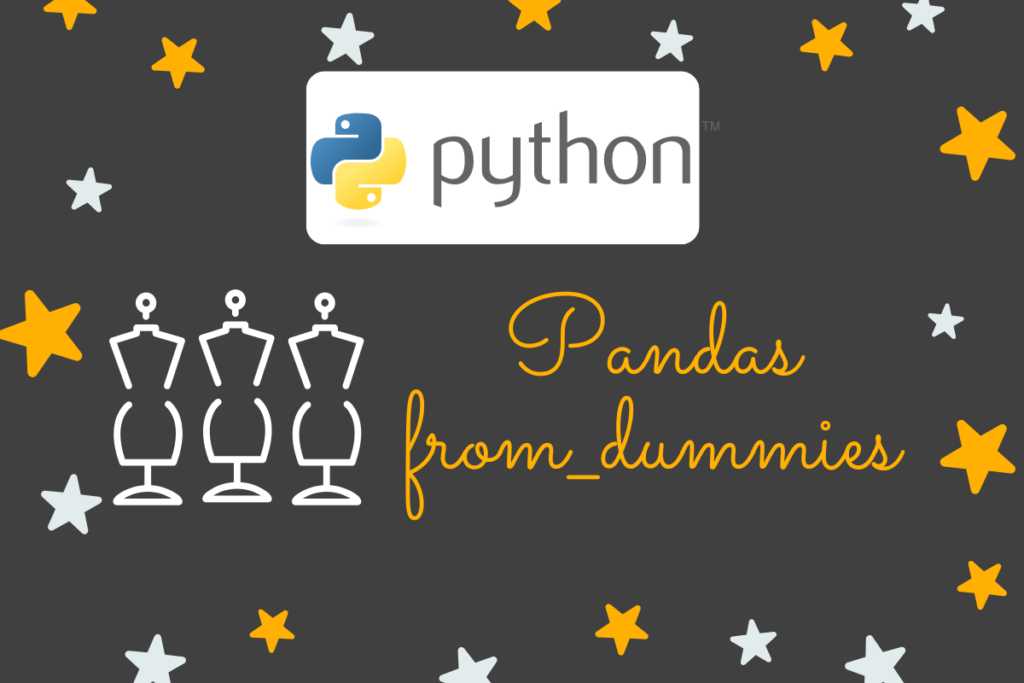# How to Use Pandas from_dummies() in Python?Machine learning brings along with it, its own peculiar means to handle data. Of the umpteen techniques available, we shall be exploring one that is used to create a categorical dataframe from a dataframe of dummy variables. The function of interest is the from_dummies( ) function within the pandas library of Python. So, let us get started by importing this library using the below code.

```import pandas as pd
```

Thereafter, we shall explore further the from_dummies( ) function through each of the following sections.

• What is a dummy variable?
• Syntax of the from_dummies( ) function
• Use cases for the from_dummies( ) function

Also read: Pandas eval(): Evaluate a Python expression as a string

## What is a dummy variable?

Dummy variables come in handy during machine learning applications. Also known as the indicator variables, these dummy variables are the binary extension to indicate the presence of a particular entity within a categorical dataset. Consider the following table with a list of responses given in the form of ‘YES’ and ‘NO’.

The above list can also be transformed in the form of dummy variables, say for instance, with those of ‘YES’, such that they return a value of ‘1’ if the entry contains ‘YES’ and ‘0’ if it contains anything else.

The same can be done for ‘NO’ too. In the cases where we only have the information tabulated on the right of the above image, then we would be needing something to get us back to the original dataframe. The from_dummies( ) function would be glad to help us with that.

## Syntax of the Pandas from_dummies( )function

Given below is the syntax of the from_dummies( ) function detailing the fundamental constituents required for its proper functioning.

```pandas.from_dummies(data, sep=None, default_category=None)
```

where,

• data – Dataframe containing the dummy variables from which the categorical dataframe is to be extracted
• sep – Indicates the type of separator used in the column names of dummy variables to differentiate between their categorical names and their corresponding prefixes. It is an optional component set to ‘None’ by default.
• default_category – Set to ‘None’ by default, this optional component is used to declare a default category value to denote the places where the dummy variables are with zeros

## Use cases for the Pandas from_dummies( ) function

In this section, we shall demonstrate the use cases of the from_dummies( ) function with a variety of dataframes. Let us get started with the one given below.

```Response = pd.DataFrame({'YES':[1, 0, 1, 1, 0, 0, 0],
'NO':[0, 1, 0, 0, 1, 1, 1]})
print(Response)
```

The above dataframe is visualised before it is transformed into its original form.

Once done, let us extract the original dataframe from the one given above using the from_dummies( ) function as shown below.

```df = pd.from_dummies(Response)
print(df)
```

Comparing this result with that of the dummy variable dataframe, one could easily infer that the entries with ‘1’ from the dummy variable dataframe are superimposed into one column with their respective categorical entry – ‘YES’ or ‘NO’. Let us now try with a use case that puts into use sep & default_category in the syntax of the from_dummies( ) function.

```Region = pd.DataFrame({'col1_Asia':[1, 0, 1, 1, 0, 0, 0],
' col2_Africa ':[0, 1, 0, 0, 1, 0, 0]})
print(Region)
```

The categorical column names contain a separator ‘_’ as seen above. So, let us tell the from_dummies( ) function this information along with the instruction to replace entries which are zero as ‘Europe’.

```df = pd.from_dummies(Region, sep=”_”, default_category=”Europe”)
print(df)
```